# What are simulation methods?

In engineering and natural sciences, many phenomena and processes are modeled by means of (partial) differential equations (PDEs). In structural analysis, they describe deformations and stresses in beams, trusses, shells, plates and so on. The exact solution of PDEs is often impossible so that solutions must be approximated using simulations methods (numerical methods). The assembly and solution of a system of equations plays a crucial role and the use of modern computers is essential for this task. The most important method for simulations in structural analysis is the finite element method (FEM). In addition, meshfree and boundary element methods provide powerful alternatives in many cases.

The development and application of simulation methods for the solution of complex engineering applications is the main focus of the Institute of Structural Analysis.

# Extended Finite Element Method (XFEM)

Many phenomena in engineering and natural sciences involve field quantities (displacements, velocities, pressure etc.) which locally feature jumps, kinks, or singularities. Examples are cracks in fracture mechanics and material interfaces in two-phase flows. The classical FEM relies on tailored meshes for the approximation of these non-smooth fields, i.e. element edges must align with the discontinuities and mesh refinements are needed near singularities. Such meshes are not easily provided, in particular in moving interface problems. In contrast, the XFEM employs simple, often structured meshes and the non-smooth solutions are considered by special enrichment functions. At the Institute of Structural Analysis, the XFEM is applied in solid mechanics (fracture, bio mechanics) and fluid mechanics (two-phase flows, fluid-structure interaction, free-surface flows).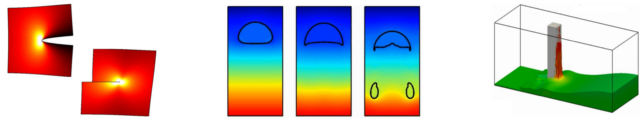# Isogeometric Analysis (IGA)

In CAD-programs, objects are defined based on non-uniform rational B-splines (NURBS). When physical quantities (such as temperature or pressure) inside these objects are of interest, the geometry is discretized by means of a mesh, typically followed by a simulation with the FEM. Unfortunately, the mesh is only an approximation of the CAD object so that important geometrical information can be lost. An example is when curved boundaries are described by piecewise linear polygons. However, it has been demonstrated that NURBS may not only be used for the geometry description in CAD but also for the approximation of the sought fields. This is called the isogeometric concept, analogously to the well-known isoparametric concept in the FEM where the geometry description and approximation are based on polynomials rather than NURBS. In the last decade, IGA has triggered a large number of research activities and can be seen as a contemporary FEM. In the context of IGA, the Institute of Structural Analysis works on trimming, collocation, and the combination with the boundary element method for various applications in solid mechanics.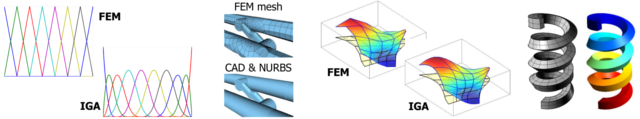# Fictitious Domain Methods

Fictitious domain methods use fixed, structured background meshes for the simulation. These meshes do not consider the geometry of the domain of interest. The boundaries of the domain cut right through the elements of the background mesh. The advantage is that no effort is needed for the mesh generation and the exact geometry description is used. However, special integration rules are needed for the cut elements and the application of boundary conditions is difficult. The Institute of Structural Analysis develops fictitious domain methods that enable higher-order accurate simulations without any meshig efforts.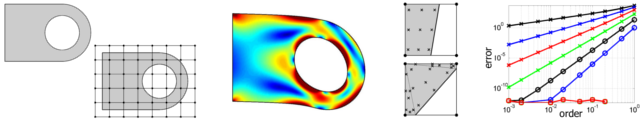# Meshfree Methods

In contrast to the classical FEM, meshfree methods only need nodes in the domain of interest - without the need for a connectivity in terms of elements (i.e., a mesh). The connectivity is determined during run-time which is important for the evaluation of the meshfree shape functions. These functions are typically generated using the moving least squares (MLS) method. When these functions are employed in the method of weighted residuals (just as in the FEM), this approach is called element-free Galerkin (EFG) method. At the Institute of Structural Analysis, meshfree methods are used in fracture and fluid mechanics.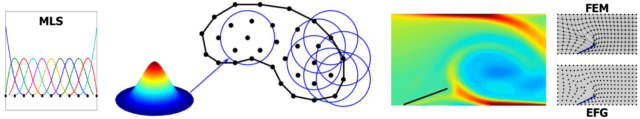# Boundary Element Method (BEM)

In the boundary element method, only the boundary of the domain of interest has to be discretized, i.e. meshed. Hence, meshing procedures are simplified and the related degrees of freedom are reduced. The method is well-suited for (semi-)infinite domains. In structure mechanics, this is for example relevant for foundations or in tunneling, in electro statics for computing electro-magnetic radiations of a device. At the Institute of Structural Analysis, a focus is on the coupling of FEM and BEM to combine the advantages of each method. In addition, isogeometric BEM formulations are of particular interest. These approaches complement the concept of isogeometric analysis very well because CAD models and BEM are based on a surface representation.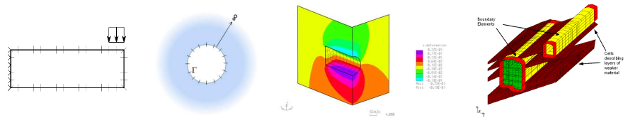The Institute of Stuctural Analysis is continuously working on advanced topics in the general FEM. Among these topics are:

• Mixed and hybrid elements
• Enhanced-assumed-strain elements and reduced-order integration
• Locking
• Adaptivity and error estimation
• Shell elements
• Iterative solvers and pre-conditioning

These topics are also relevant in the context of non-linear models, especially in plasticity and finite deformations.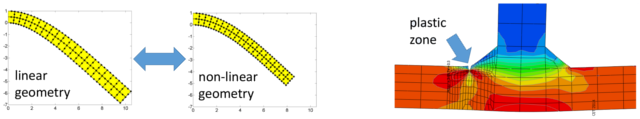Pics: ©Fries - TU Graz/IFB